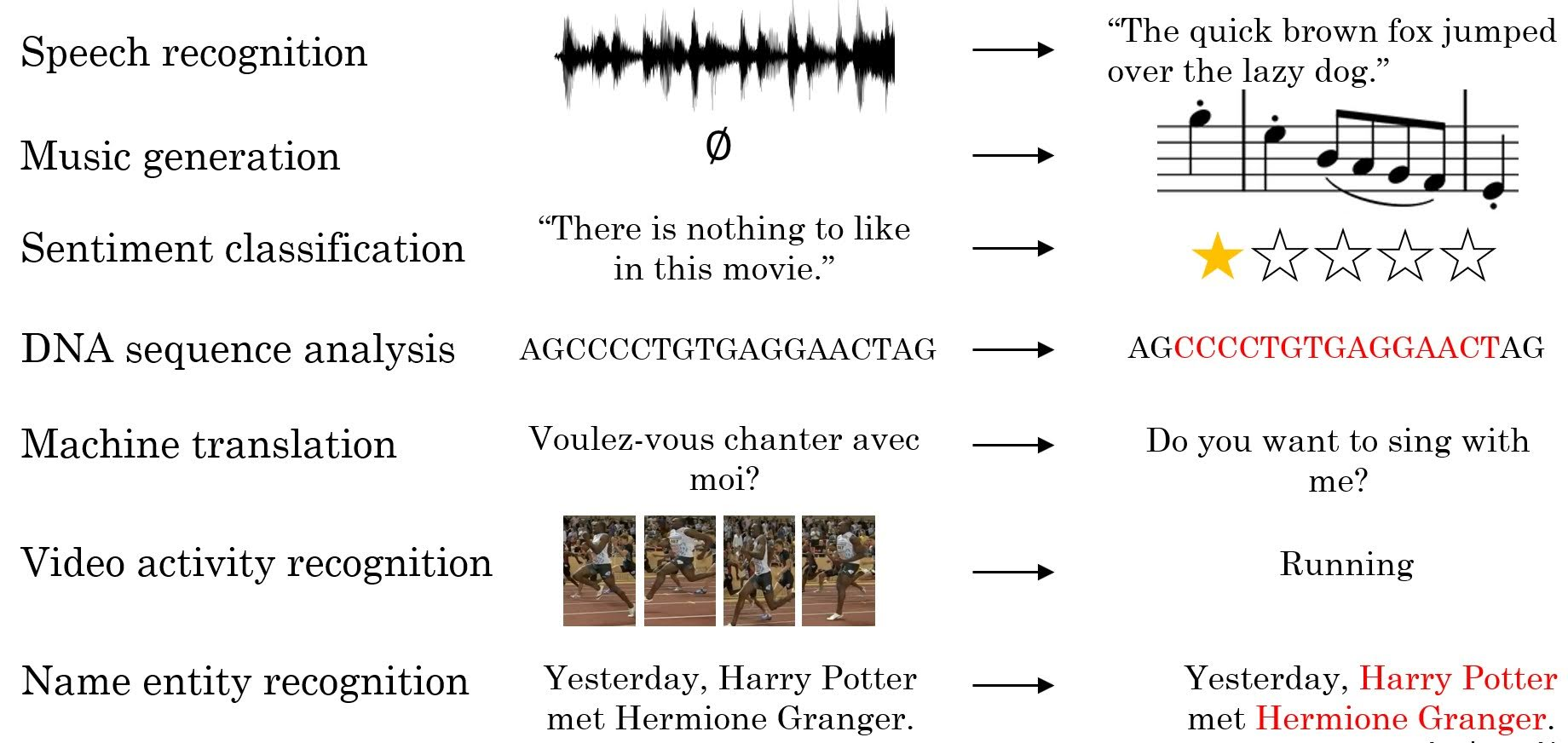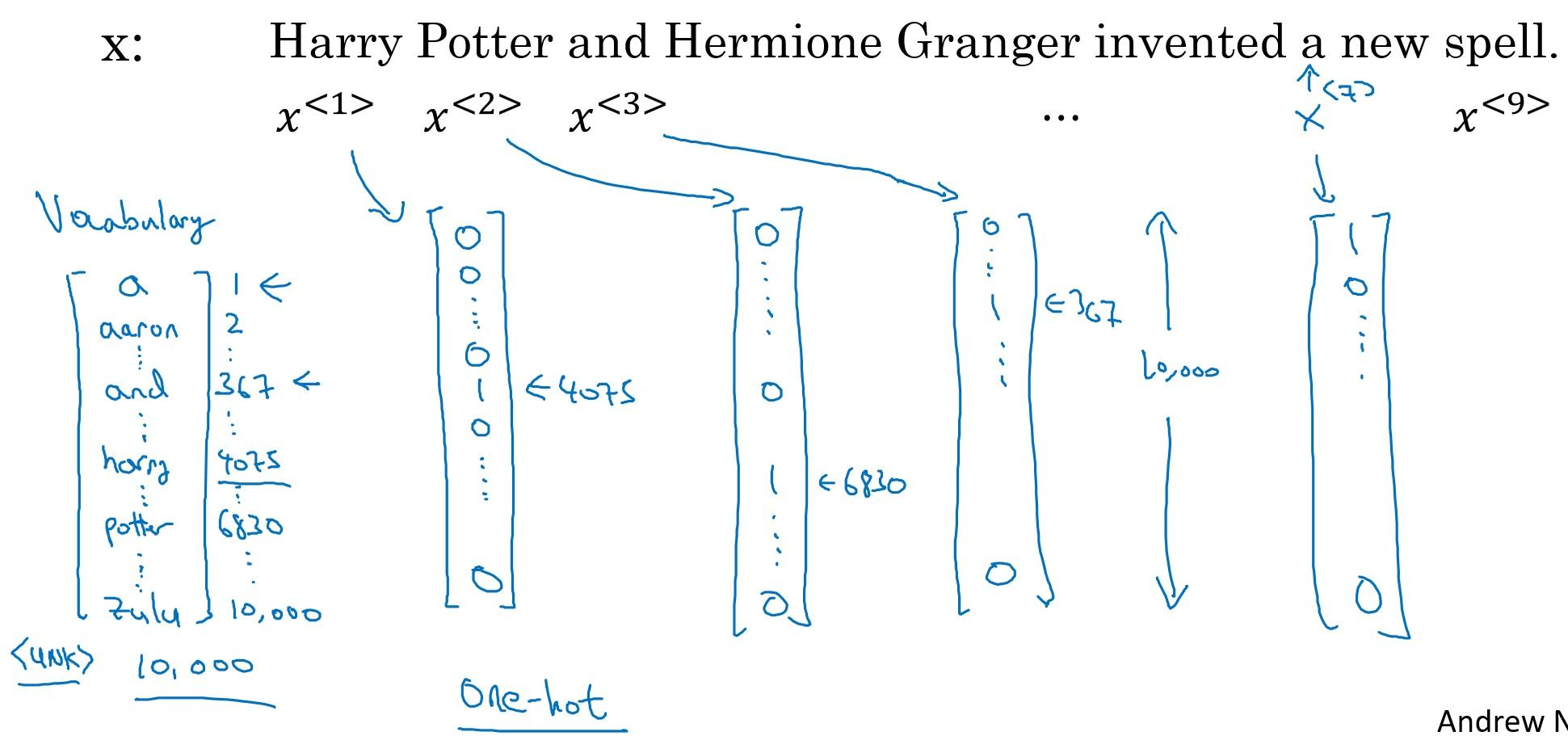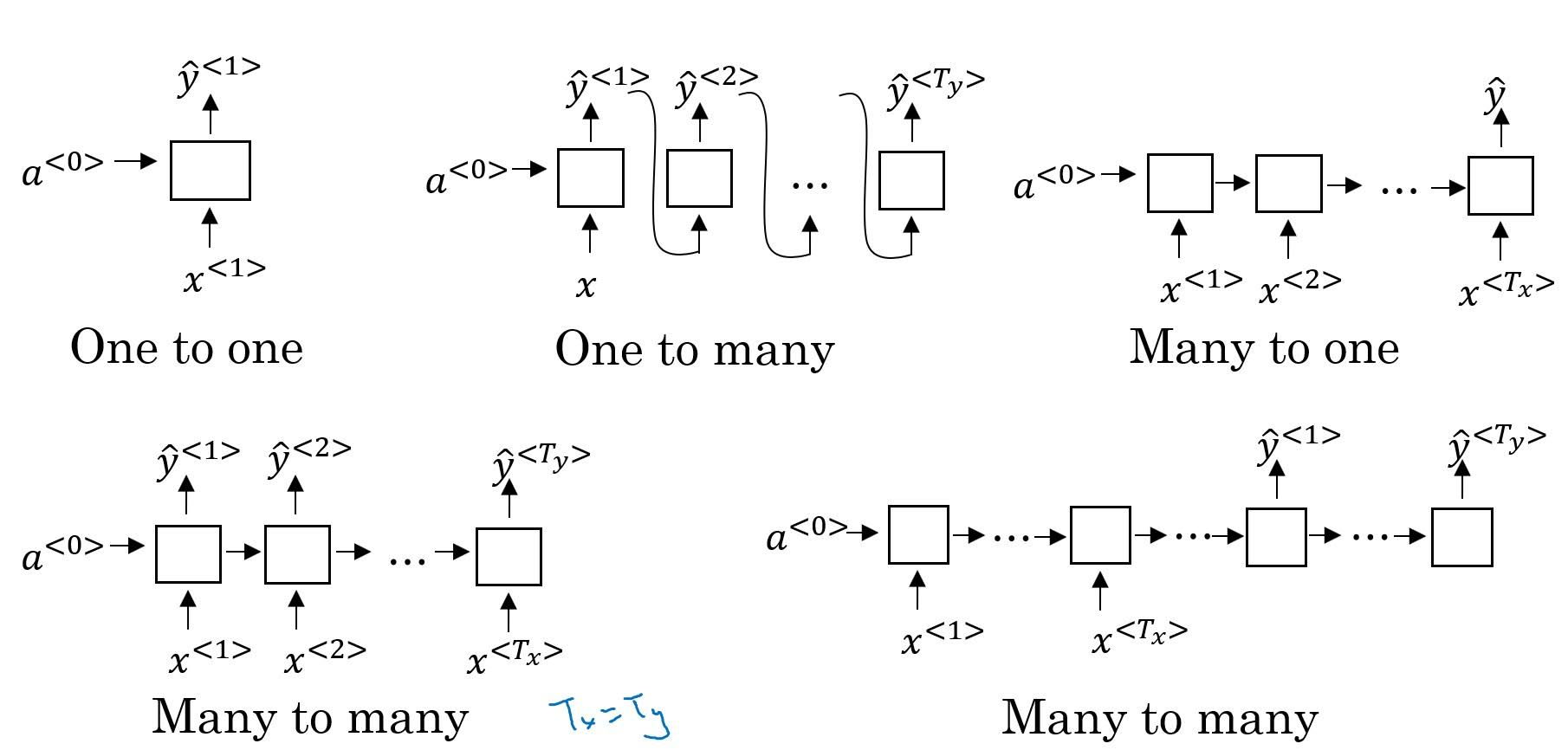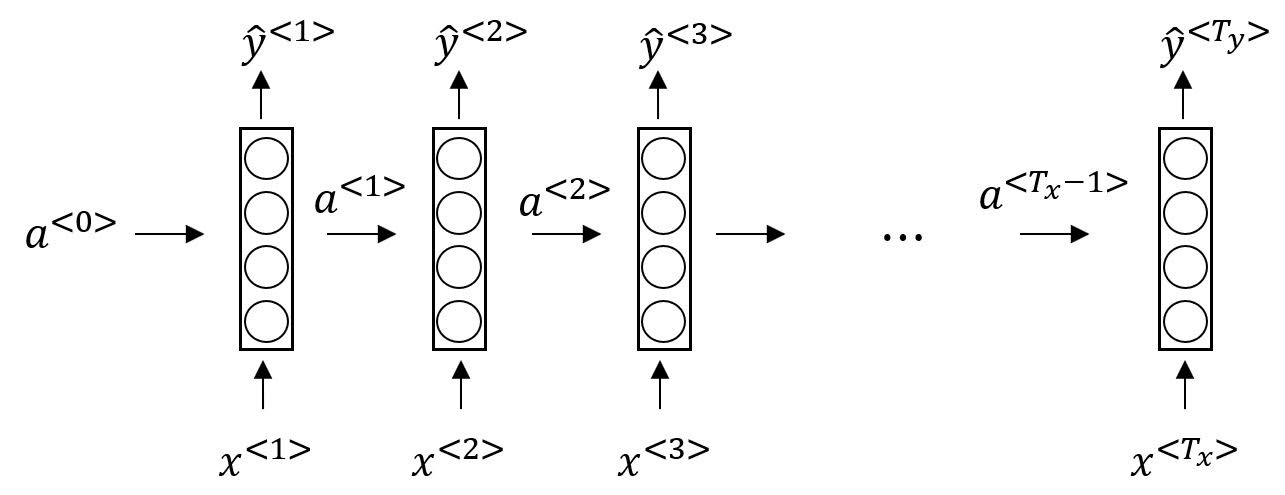# What is a Recurrent Neural Network?

Categories:

Updated:

A recurrent neural network (RNN) is a class of artificial neural network where connections between nodes form a directed graph along a sequence. This allows it to exhibit temporal dynamic behavior for a time sequence.

So, RNN is appled to sequence data like text, audio, video, DNA, etc.

# Examples of Where RNNs Apply

1. Speech Recognition: with input audio, output transcription
2. Music generation: generate music
3. Sentiment Classification: from a movie comment, output rating
4. DNA Sequence Analysis: from DNA sequencee, determine the order of nucleotides in DNA
5. Machine Translation: translate text from one language to another
6. Video Activity Recognition: from a video of a person moving, determine if that person is running
7. Name Entity Recognition: from a text, extract names# How do we Represent Words?

How do we handle text? We should make words understandable to RNN. One simple way to do this is to vectorize words.

For example, let’s say we have dictionary composed of 10000 words. Then we can represent each word in the dictionary as a one-hot vector. If ‘spell’ is the 7498th word in the dictionary, the word ‘spell’ can be represented as a 10000-long vector composed of 0s except 7498th element which would be 1.

After we’ve represented words with vectors, we can represent a sentence with sequential vectors.# Different Types of RNNs‘One to many’ can be applied to music generation, ‘many to one’ to sentiment classification, ‘many to many’ to name entity recognition, ‘many to many(encoding-decoding)’ to machine translation.

# Forward Propagation in Simple Many to Many RNN

In RNN, activation of a $t$th sequence influences activation of a $t+1$th sequence.There are two outputs per hidden layer; $a$ and $\hat{y}$.

### 1. How to compute $a$

The above formula can be simplified as follows;

where $W_a$ is a horizontal stack of $W_{aa}$ and $W_{ax}$ , and $[a^{}, x^{}]$ is a vertical stack of $a^{}$ and $x^{}$ .

# Why RNN?

When dealing with sequence data, why do we use RNN, not standard network ?

1. Inputs and outputs can be different lengths for different examples
2. Can share features learned across different positions of text

Categories: